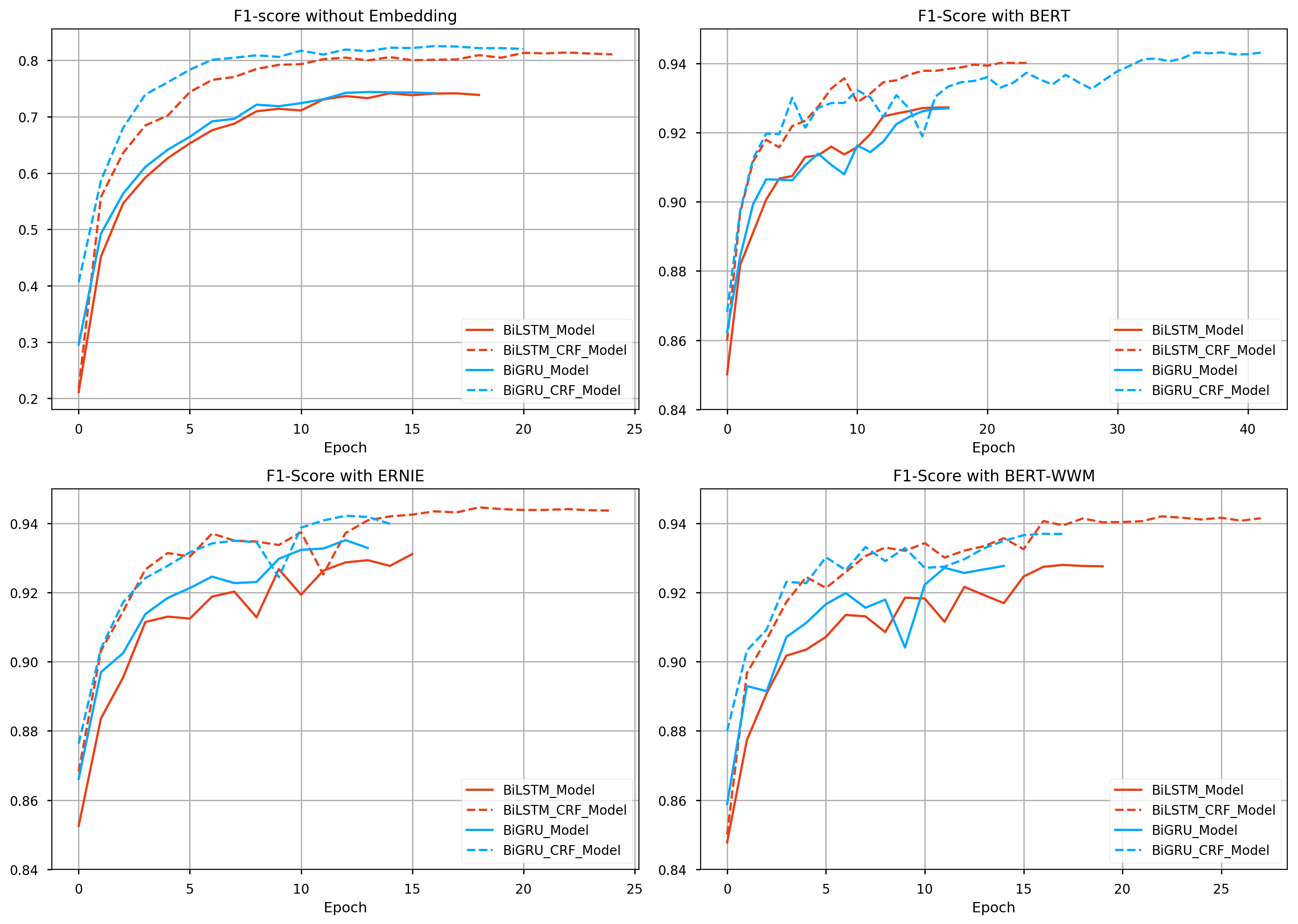# Text Labeling Model¶

Kashgari provides several models for text labeling, All labeling models inherit from the `BaseLabelingModel`. You could easily switch from one model to another just by changing one line of code.

Name Info
CNN_LSTM_Model
BiLSTM_Model
BiLSTM_CRF_Model
BiGRU_Model
BiGRU_CRF_Model

## Train basic NER model¶

Kashgari provices basic NER corpus for expirement. You could also use your corpus in any language for training.

```# Load build-in corpus.
## For Chinese
from kashgari.corpus import ChineseDailyNerCorpus

## For English
from kashgari.corpus import CONLL2003ENCorpus

# Or use your own corpus
train_x = [['Hello', 'world'], ['Hello', 'Kashgari'], ['I', 'love', 'Beijing']]
train_y = [['O', 'O'], ['O', 'B-PER'], ['O', 'B-LOC']]

valid_x, valid_y = train_x, train_y
test_x, test_x = train_x, train_y
```

Or use your own corpus, it needs to be tokenized like this.

```>>> print(train_x)
['海', '钓', '比', '赛', '地', '点', '在', '厦', '门', '与', '金', '门', '之', '间', '的', '海', '域', '。']

>>> print(train_y)
['O', 'O', 'O', 'O', 'O', 'O', 'O', 'B-LOC', 'I-LOC', 'O', 'B-LOC', 'I-LOC', 'O', 'O', 'O', 'O', 'O', 'O']
```

Then train our first model. All models provided some APIs, so you could use any labeling model here.

```import kashgari

model = BLSTMModel()
model.fit(train_x, train_y, valid_x, valid_y)

# Evaluate the model

model.evaluate(test_x, test_y)

# Model data will save to `saved_ner_model` folder
model.save('saved_ner_model')

# To continue training, compile the newly loaded model first
model.fit(train_x, train_y, valid_x, valid_y)
```

That’s all your need to do. Easy right.

## Sequence labeling with transfer learning¶

Kashgari provides varies Language model Embeddings for transfer learning. Here is the example for BERT Embedding.

```import kashgari
from kashgari.embeddings import BERTEmbedding

bert_embed = BERTEmbedding('<PRE_TRAINED_BERT_MODEL_FOLDER>',
sequence_length=100)
model = BLSTMModel(bert_embed)
model.fit(train_x, train_y, valid_x, valid_y)
```

You could replace bert_embedding with any Embedding class in `kashgari.embeddings`. More info about Embedding: LINK THIS.

You could easily change model’s hyper-parameters. For example, we change the lstm unit in `BLSTMModel` from 128 to 32.

```from kashgari.tasks.labeling import BLSTMModel

hyper = BLSTMModel.get_default_hyper_parameters()
print(hyper)
# {'layer_blstm': {'units': 128, 'return_sequences': True}, 'layer_dropout': {'rate': 0.4}, 'layer_time_distributed': {}, 'layer_activation': {'activation': 'softmax'}}

hyper['layer_blstm']['units'] = 32

model = BLSTMModel(hyper_parameters=hyper)
```

## Use custom optimizer¶

```from kashgari.corpus import SMP2018ECDTCorpus
# Remember to import kashgari before than RAdam

model = BiLSTM_Model()
# This step will build token dict, label dict and model structure
model.build_model(train_x, train_y, valid_x, valid_y)
# Compile model with custom optimizer, you can also customize loss and metrics.
model.compile_model(optimizer=optimizer)

# Train model
model.fit(train_x, train_y, valid_x, valid_y)
```

## Use callbacks¶

Kashgari is based on keras so that you could use all of the tf.keras callbacks directly with Kashgari model. For example, here is how to visualize training with tensorboard.

```from tensorflow.python import keras
from kashgari.callbacks import EvalCallBack

model = BLSTMModel()

tf_board_callback = keras.callbacks.TensorBoard(log_dir='./logs', update_freq=1000)

# Build-in callback for print precision, recall and f1 at every epoch step
eval_callback = EvalCallBack(kash_model=model,
valid_x=valid_x,
valid_y=valid_y,
step=5)

model.fit(train_x,
train_y,
valid_x,
valid_y,
batch_size=100,
callbacks=[eval_callback, tf_board_callback])
```

It is very easy and straightforward to build your own customized model, just inherit the `BaseLabelingModel` and implement the `get_default_hyper_parameters()` function and `build_model_arc()` function.

```from typing import Dict, Any

from tensorflow import keras

from kashgari.layers import L

import logging
logging.basicConfig(level='DEBUG')

class DoubleBLSTMModel(BaseLabelingModel):
"""Bidirectional LSTM Sequence Labeling Model"""

@classmethod
def get_default_hyper_parameters(cls) -> Dict[str, Dict[str, Any]]:
"""
Get hyper parameters of model
Returns:
hyper parameters dict
"""
return {
'layer_blstm1': {
'units': 128,
'return_sequences': True
},
'layer_blstm2': {
'units': 128,
'return_sequences': True
},
'layer_dropout': {
'rate': 0.4
},
'layer_time_distributed': {},
'layer_activation': {
'activation': 'softmax'
}
}

def build_model_arc(self):
"""
build model architectural
"""
output_dim = len(self.processor.label2idx)
config = self.hyper_parameters
embed_model = self.embedding.embed_model

layer_blstm1 = L.Bidirectional(L.LSTM(**config['layer_blstm1']),
name='layer_blstm1')
layer_blstm2 = L.Bidirectional(L.LSTM(**config['layer_blstm2']),
name='layer_blstm2')

layer_dropout = L.Dropout(**config['layer_dropout'],
name='layer_dropout')

layer_time_distributed = L.TimeDistributed(L.Dense(output_dim,
**config['layer_time_distributed']),
name='layer_time_distributed')
layer_activation = L.Activation(**config['layer_activation'])

# Define tensor flow
tensor = layer_blstm1(embed_model.output)
tensor = layer_blstm2(tensor)
tensor = layer_dropout(tensor)
tensor = layer_time_distributed(tensor)
output_tensor = layer_activation(tensor)

# Init model
self.tf_model = keras.Model(embed_model.inputs, output_tensor)

model = DoubleBLSTMModel()
model.fit(train_x, train_y, valid_x, valid_y)
```

## Speed up using CuDNN cell¶

You can speed up training and inferencing process using CuDNN cell. CuDNNLSTM and CuDNNGRU layers are much faster than LSTM and GRU layer, but they must be used on GPU. If you want to train on GPU and inferencing on CPU, you cannot use CuDNN cells.

```# Enable use cudnn cell
kashgari.config.use_cudnn_cell = True
```

## Performance report¶

Available model list, matrics based on this training:

• corpus: ChineseDailyNerCorpus
• epochs: 50 epochs with callbacks
• batch_size: 64
• T4 GPU / 2 CPU / 30 GB on openbayes
```early_stop = keras.callbacks.EarlyStopping(patience=10)
reduse_lr_callback = keras.callbacks.ReduceLROnPlateau(factor=0.1, patience=5)
```
Name Embedding F1 Score Epoch Time Non Trainable params Trainable params
BiLSTM_Model Random Init 0.74147 9.5s 0 558176
BiLSTM_CRF_Model Random Init 0.81378 123.0s 0 573168
BiGRU_Model Random Init 0.74375 9.7s 0 499296
BiGRU_CRF_Model Random Init 0.82516 120.7s 0 514288
BiLSTM_Model BERT 0.92727 183.0s 101360640 3280904
BiLSTM_CRF_Model BERT 0.94013 265.0s 101360640 3295896
BiGRU_Model BERT 0.92700 180.4s 101360640 2461192
BiGRU_CRF_Model BERT 0.94319 263.4s 101360640 2476184
BiLSTM_Model ERNIE 0.93109 167.6s 98958336 3280904
BiLSTM_CRF_Model ERNIE 0.94460 250.6s 98958336 3295896
BiGRU_Model ERNIE 0.93512 165.7s 98958336 2461192
BiGRU_CRF_Model ERNIE 0.94218 250.4s 98958336 2476184